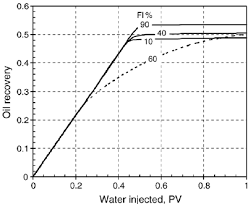How to Calculate and Solve for the Water – Oil Ratio of a Reservoir Fluid Flow | The Calculator EncyclopediaThe above image represents Water – Oil Ratio.

To compute for the water – oil ratio, six essential parameters are needed and these parameters are o)Oil Viscosity, (μw)Water Viscosity, (krw)Relative Permeability to Water, (kro)Relative Permeability to Oil, (Bw)Water Formation Volume Factor and (Bo)Oil Formation Volume Factor.

The formula for calculating the water – oil ratio:

WOR = [krw / kro] [μoBo  / μwBw ]

Where;

WOR = Water Oil Ratio
μo = Oil Viscosity
μw = Water Viscosity
krw = Relative Permeability to Water
kro = Relative Permeability to Oil
Bw = Water Formation Volume Factor
Bo = Oil Formation Volume Factor

Let’s solve an example;
Find the water – oil ratio when the oil viscosity is 9, water viscosity is 11 with a relative permeability to water of 21, relative permeability to oil of 7 and a water formation volume factor of 14 with oil formation volume factor of 27.

This implies that;

μo = Oil Viscosity = 9
μw = Water Viscosity = 11
krw = Relative Permeability to Water = 21
kro = Relative Permeability to Oil = 7
Bw = Water Formation Volume Factor = 14
Bo = Oil Formation Volume Factor = 27

WOR = [krw / kro] [μoBo  / μwBw ]
WOR = [21/7] [9 x 27 /11 x 14]
WOR = [21/7] [243/154]
WOR = [21/7] [1.5779]
WOR =  [1.5779]
WOR = 4.73

Therefore, the Water – Oil Ratio is 4.73.